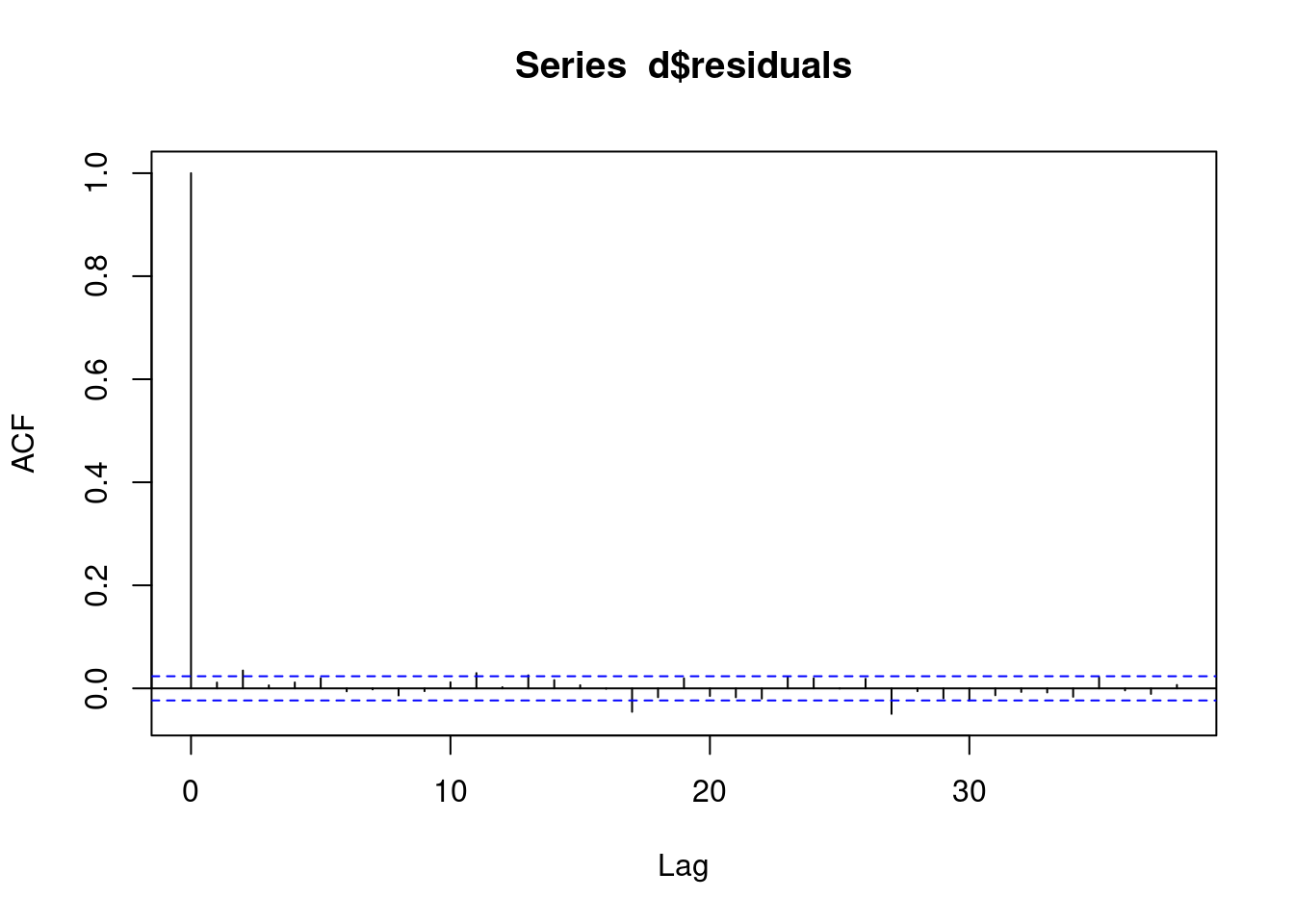## 2.1 Aim

We are given a dataset containing daily counts of diseases from one geographical area. We want to identify:

• Does seasonality exist?
• If seasonality exists, when are the high/low seasons?
• Is there a general yearly trend (i.e. increasing or decreasing from year to year?)
• Is daily rainfall associated with the number of cases?

## 2.2 Creating the data

The data for this chapter is available at: https://www.csids.no/longitudinal-analysis-for-surveillance/data/chapter_2.csv

``````# R CODE

library(data.table)
library(ggplot2)
set.seed(4)

AMPLITUDE <- 1.5
SEASONAL_HORIZONTAL_SHIFT <- 20

d <- data.table(date=seq.Date(
from=as.Date("2000-01-01"),
to=as.Date("2018-12-31"),
by=1))
d[,year:=as.numeric(format.Date(date,"%G"))]
d[,week:=as.numeric(format.Date(date,"%V"))]
d[,month:=as.numeric(format.Date(date,"%m"))]
d[,yearMinus2000:=year-2000]
d[,dailyrainfall:=runif(.N, min=0, max=10)]

d[,dayOfYear:=as.numeric(format.Date(date,"%j"))]
d[,seasonalEffect:=sin(2*pi*(dayOfYear-SEASONAL_HORIZONTAL_SHIFT)/365)]
d[,mu := exp(0.1 + yearMinus2000*0.1 + seasonalEffect*AMPLITUDE)]
d[,y:=rpois(.N,mu)]``````

## 2.3 True data

Here we show the true data, and note that there is an increasing annual trend (the data gets higher as time goes on) and there is a seasonal pattern (one peak/trough per year)

``````q <- ggplot(d,aes(x=date))
q <- q + geom_point(mapping=aes(y=y))
q <- q + geom_line(mapping=aes(y=mu),colour="red")
q``````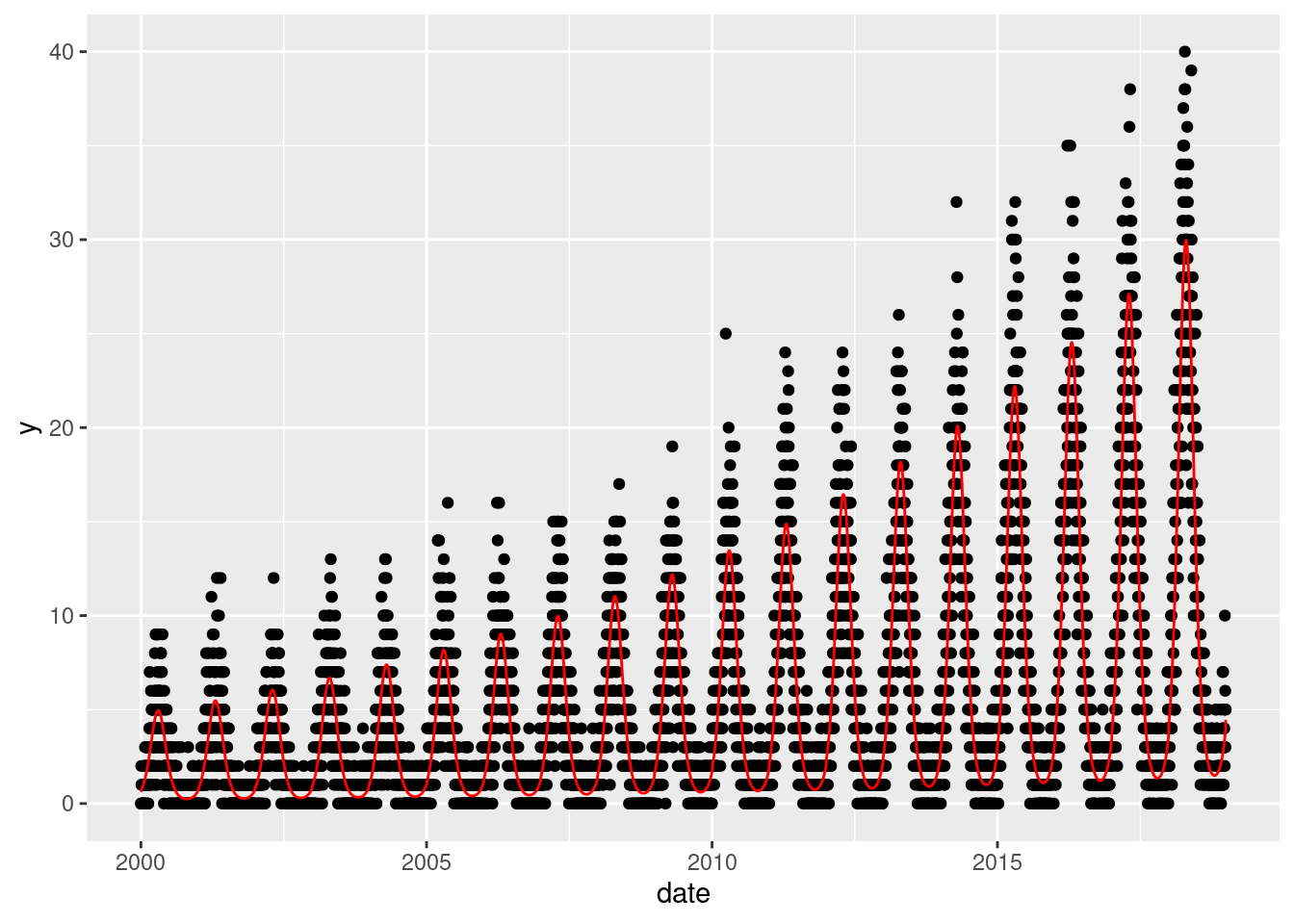## 2.4 Investigation

Pretending we have no prior knowledge of our dataset, we display the data for few years and see a clear seasonal trend

``````q <- ggplot(d[year %in% c(2005:2010)],aes(x=dayOfYear,y=y))
q <- q + facet_wrap(~year)
q <- q + geom_point()
q <- q + stat_smooth(colour="red")
q``````
```geom_smooth()` using method = 'loess' and formula 'y ~ x'``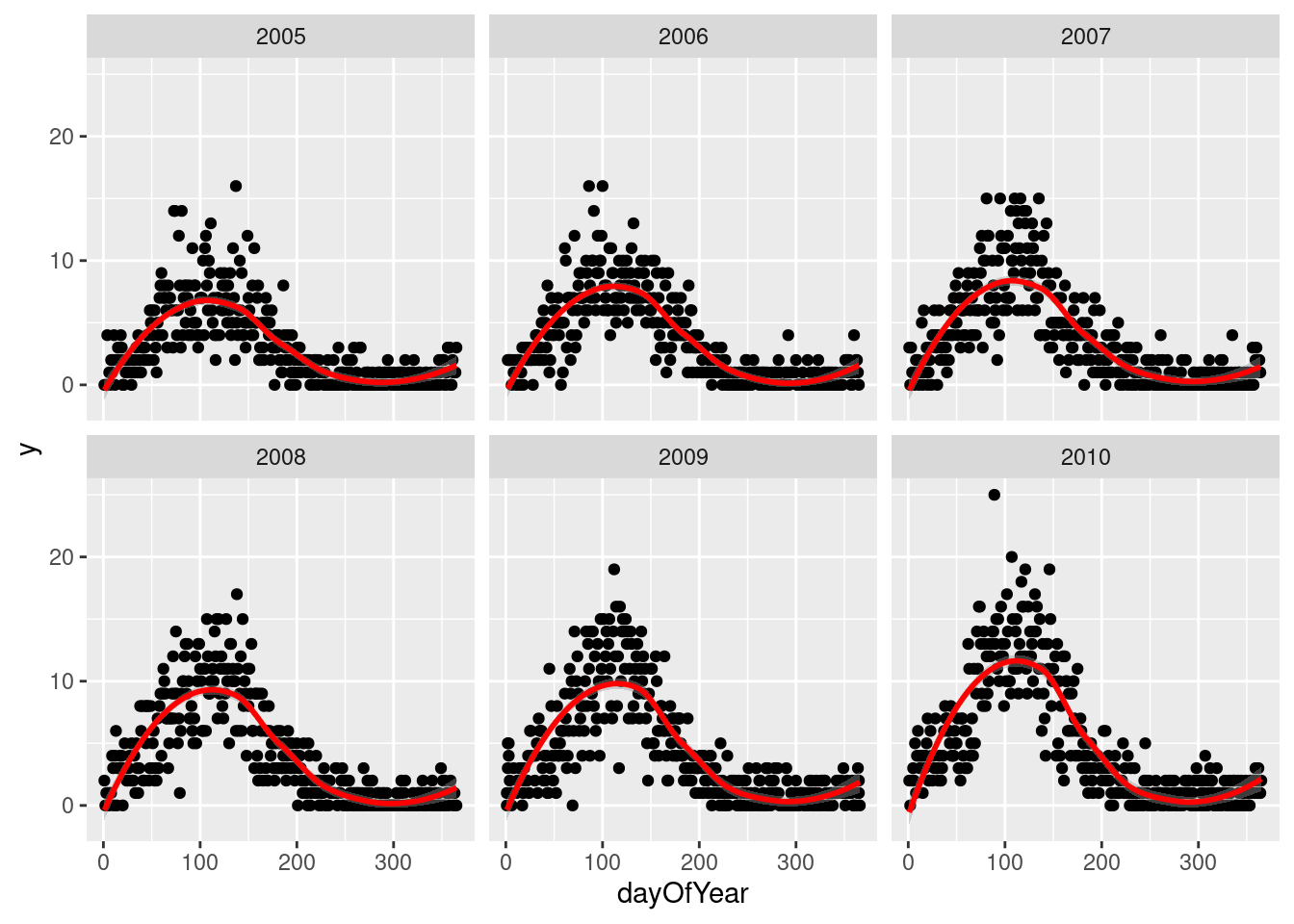## 2.5 Seasonality

If we want to investigate the seasonality of our data, and identify when are the peaks and troughs, we have a few ways to approach this.

Non-parametric approaches are flexible and easy to implement, but they can lack power and be hard to interpret:

• Create a categorical variable for the seasons (e.g. `spring`, `summer`, `autumn`, `winter`) and include this in the regression model
• Create a categorical variable for the months (e.g. `Jan`, `Feb`, …, `Dec`) and include this in the regression model

Parametric approaches are more powerful but require more effort:

• Identify the periodicity of the seasonality (how many days between peaks?)
• Using trigonometry, transform `day of year` into variables that appropriately model the observed periodicity
• Obtain coefficient estimates
• Back-transform these estimates into human-understandable values (day of peak, day of trough)

The non-parametric approaches are simple and we will therefore not cover them in this course. We will briefly examine the parametric approach.

NOTE: You don’t always have to investigate seasonality! It depends entirely on what the purpose of your analysis is!

The Lomb-Scargle Periodogram shows a clear seasonality with a period of 365 days.

``````// STATA CODE STARTS
insheet using "chapter_3.csv", clear

sort date
gen time=_n
tsset time, daily

wntestb y

cumsp y, gen(cumulative_spec_dist)
gen period=_N/_n

browse cumulative_spec_dist period
// STATA CODE ENDS``````
``````# R CODE
lomb::lsp(d\$y,from=100,to=500,ofac=1,type="period")``````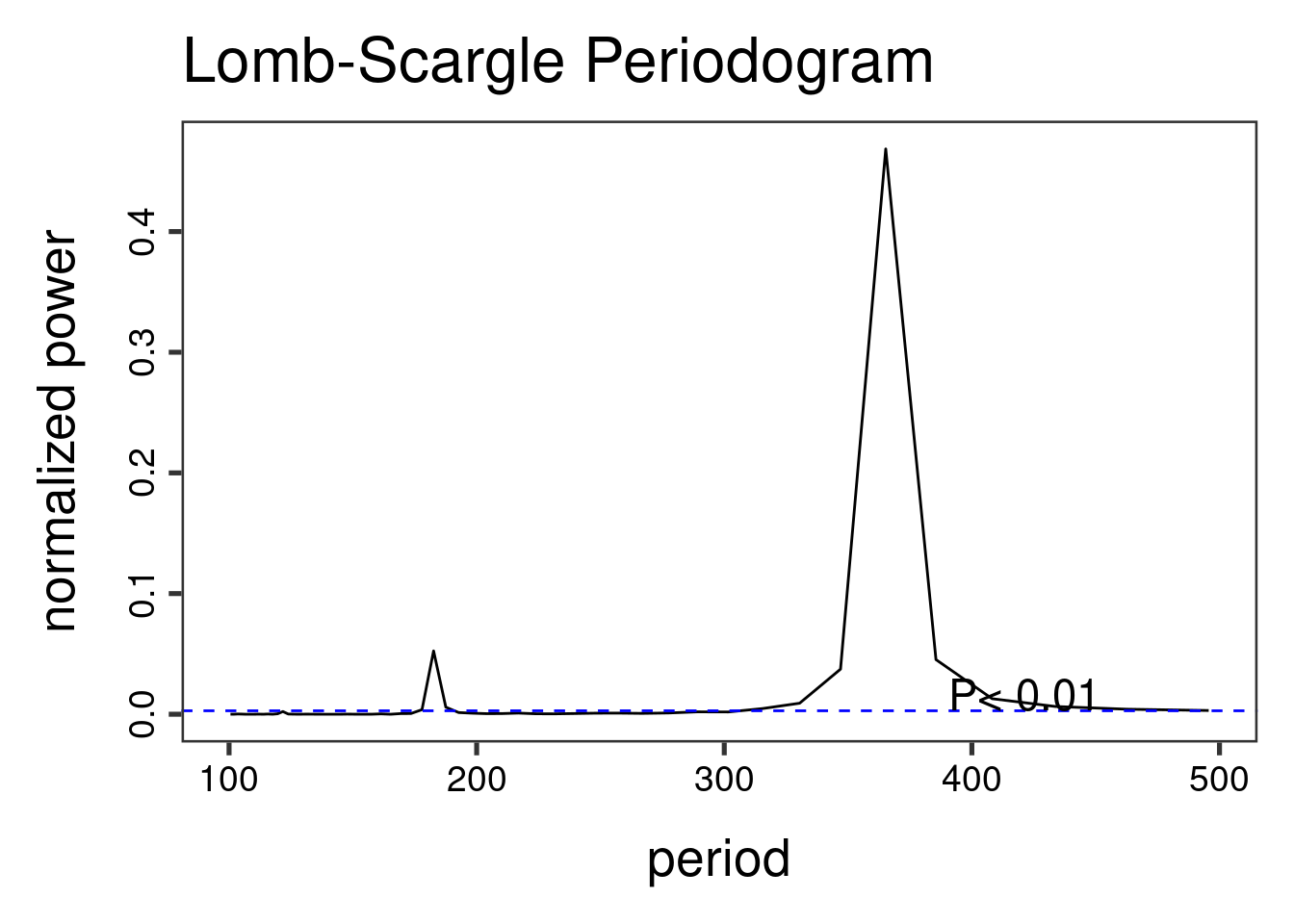We then generate two new variables `cos365` and `sin365` and perform a likelihood ratio test to see if they are significant or not. This is done with two simple poisson regressions.

When we do not have autocorrelation, we can use the `glm` function in R and in STATA. Note that it is very important to specify the `family` (as this is how we differentiate between linear/logistic/poisson regressions).

``````// STATA CODE STARTS
gen cos365=cos(dayofyear*2*_pi/365)
gen sin365=sin(dayofyear*2*_pi/365)

glm y yearminus2000 dailyrainfall, family(poisson)
estimates store m1
glm y yearminus2000 dailyrainfall cos365 sin365, family(poisson)
estimates store m2

predict resid, anscombe

lrtest m1 m2
// STATA CODE ENDS``````
``````# R CODE
d[,cos365:=cos(dayOfYear*2*pi/365)]
d[,sin365:=sin(dayOfYear*2*pi/365)]

fit0 <- glm(y~yearMinus2000 + dailyrainfall, data=d, family=poisson())
fit1 <- glm(y~yearMinus2000 + dailyrainfall + sin365 + cos365, data=d, family=poisson())

print(lmtest::lrtest(fit0, fit1))``````
``````Likelihood ratio test

Model 1: y ~ yearMinus2000 + dailyrainfall
Model 2: y ~ yearMinus2000 + dailyrainfall + sin365 + cos365
#Df LogLik Df Chisq Pr(>Chisq)
1   3 -26904
2   5 -12892  2 28024  < 2.2e-16 ***
---
Signif. codes:  0 '***' 0.001 '**' 0.01 '*' 0.05 '.' 0.1 ' ' 1``````

We see that the likelihood ratio test for `sin365` and `cos365` was significant, meaning that there is significant seasonality with a 365 day periodicity in our data (which we already strongly suspected due to the periodogram).

We can now run/look at the results of our main regression.

``print(summary(fit1))``
``````
Call:
glm(formula = y ~ yearMinus2000 + dailyrainfall + sin365 + cos365,
family = poisson(), data = d)

Deviance Residuals:
Min       1Q   Median       3Q      Max
-4.0676  -0.9229  -0.1170   0.5861   3.4103

Coefficients:
Estimate Std. Error z value Pr(>|z|)
(Intercept)    0.0887436  0.0176742   5.021 5.14e-07 ***
yearMinus2000  0.1016117  0.0010525  96.539  < 2e-16 ***
dailyrainfall  0.0002287  0.0018476   0.124    0.901
sin365         1.3972586  0.0103200 135.393  < 2e-16 ***
cos365        -0.5035265  0.0086308 -58.341  < 2e-16 ***
---
Signif. codes:  0 '***' 0.001 '**' 0.01 '*' 0.05 '.' 0.1 ' ' 1

(Dispersion parameter for poisson family taken to be 1)

Null deviance: 45536.8  on 6939  degrees of freedom
Residual deviance:  7328.5  on 6935  degrees of freedom
AIC: 25794

Number of Fisher Scoring iterations: 5``````

We also see that the (significant!) coefficient for `year` is `0.1` which means that for each additional year, the outcome increases by `exp(0.1)=1.11`. We also see that the coefficient for `dailyrainfall` was not significant, which means that we did not find a significant association between the outcome and `dailyrainfall`.

NOTE: See that this is basically the same as a normal regression.

Through the likelihood ratio test we saw a clear significant seasonal effect. We can now use trigonometry to back-calculate the amplitude and location of peak/troughs from the `cos365` and `sin365` estimates:

``````b1 <- 1.428417 # sin coefficient
b2 <- -0.512912 # cos coefficient
amplitude <- sqrt(b1^2 + b2^2)
p <- atan(b1/b2) * 365/2/pi
if (p > 0) {
peak <- p
trough <- p + 365/2
} else {
peak <- p + 365/2
trough <- p + 365
}
if (b1 < 0) {
g <- peak
peak <- trough
trough <- g
}
print(sprintf("amplitude is estimated as %s, peak is estimated as %s, trough is estimated as %s",round(amplitude,2),round(peak),round(trough)))``````
`` "amplitude is estimated as 1.52, peak is estimated as 111, trough is estimated as 294"``
``print(sprintf("true values are: amplitude: %s, peak: %s, trough: %s",round(AMPLITUDE,2),round(365/4+SEASONAL_HORIZONTAL_SHIFT),round(3*365/4+SEASONAL_HORIZONTAL_SHIFT)))``
`` "true values are: amplitude: 1.5, peak: 111, trough: 294"``

NOTE: An amplitude of 1.5 means that when comparing the average time of year to the peak, the peak is expected to be `exp(1.5)=4.5` times higher than average. We take the exponential because we have run a poisson regression (so think incident rate ratio).

We now investigate our residuals to determine if we have a good fit:

``````d[,residuals:=residuals(fit1, type = "response")]
d[,predicted:=predict(fit1, type = "response")]
q <- ggplot(d,aes(x=predicted,y=residuals))
q <- q + geom_point()
q <- q + stat_smooth(colour="red")
q``````
```geom_smooth()` using method = 'gam' and formula 'y ~ s(x, bs = "cs")'``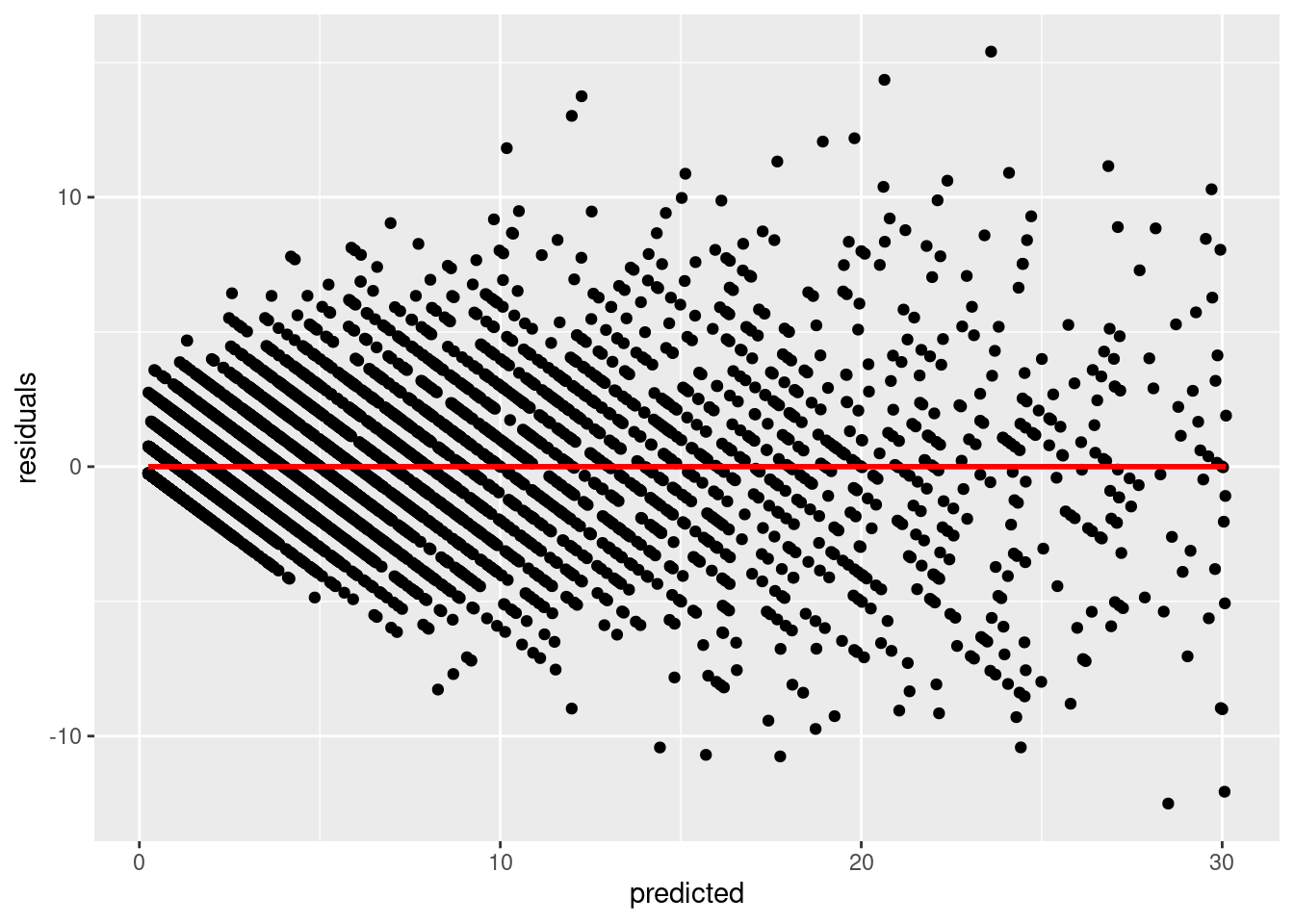We check the `pacf` of the residuals to ensure that it is not `AR`. If we observe `AR` in our residuals, then this model was not appropriate and we need to use a different model.

``````// STATA CODE STARTS
pac resid
// STATA CODE ENDS``````
``````# R CODE
# this is for AR
pacf(d\$residuals)``````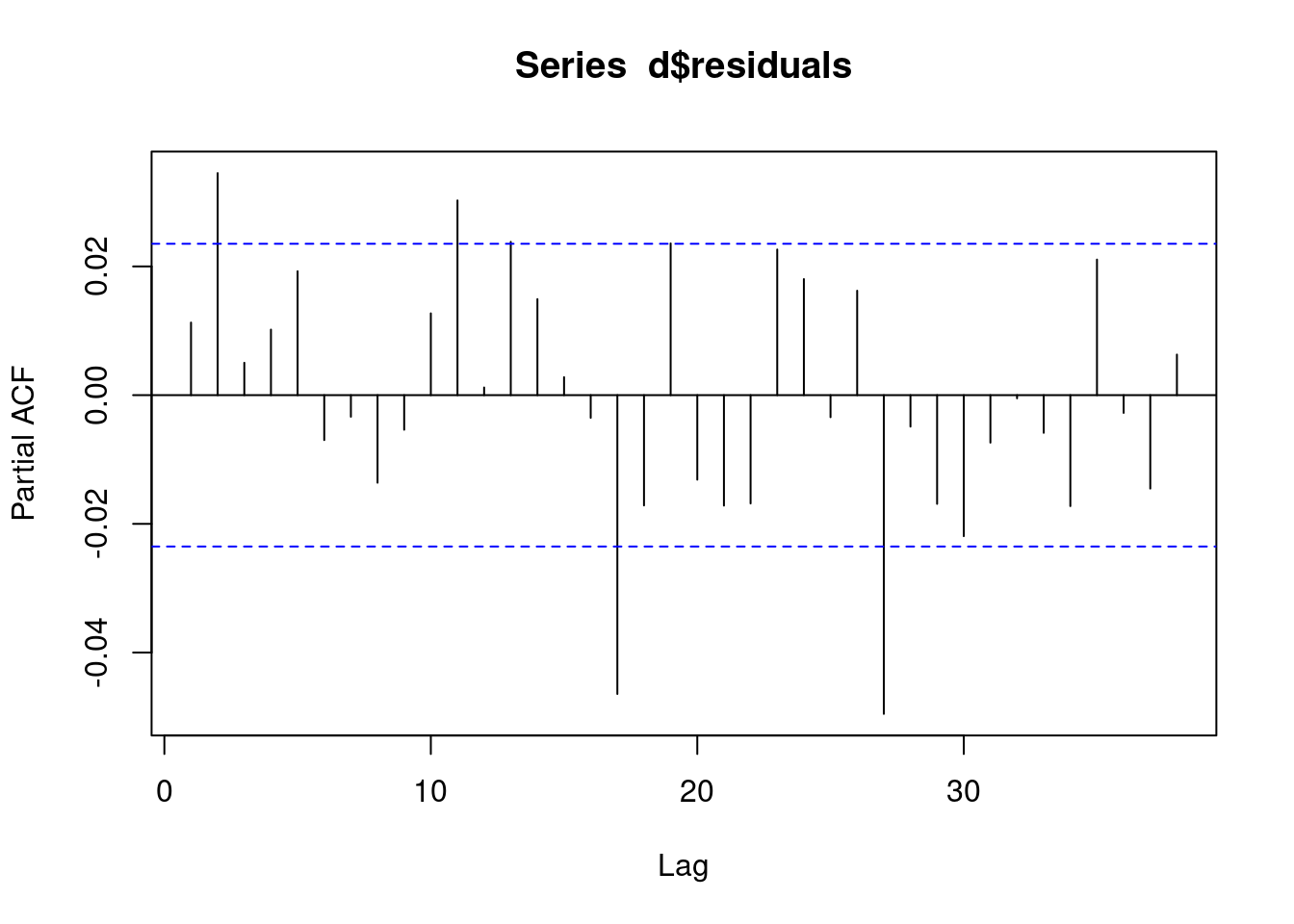We check the `acf` of the residuals to ensure that it is not `MA`. If we observe `MA` in our residuals, then this model was not appropriate and we need to use a different model.

``````// STATA CODE STARTS
ac resid
// STATA CODE ENDS``````
``````# R CODE
# this is for MA
acf(d\$residuals)``````